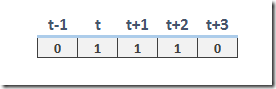## Wednesday, June 14, 2017

### Modeling production runs with length exactly three

In (1) the question was posed how to model production runs that have an exact length. Although the question was asked in terms of integer variables, this is much easier to deal with when we have binary variables. Let’s introduce a binary variable:

 $x_{t} = \begin{cases}1 & \text{when the unit x is turned on in period t}\\0 &\text{otherwise}\end{cases}$

The question was formulated as an implication:

 $x_t=1 \implies x_{t+1}=x_{t+2}=1$

This implication is not 100% correct: it would turn on $$x$$ forever. But we understand what the poster meant, if a unit is turned on, it should stay on for exactly three periods.This condition can be more correctly stated as the implication:

 $x_{t-1}=0 \text{ and } x_{t}=1 \implies x_{t+1}=x_{t+2}=1 \text{ and } x_{t+3}=0$

We can make linear inequalities out of this as follows:

 \bbox[lightcyan,10px,border:3px solid darkblue]{\begin{align}&x_{t+1}\ge x_{t}-x_{t-1}\\&x_{t+2}\ge x_{t}-x_{t-1}\\&1-x_{t+3}\ge x_{t}-x_{t-1}\end{align}}

If we want to model the condition “a unit should stay on for at least three periods”, we can drop the last constraint and just keep:

 \begin{align}&x_{t+1}\ge x_{t}-x_{t-1}\\&x_{t+2}\ge x_{t}-x_{t-1}\end{align}

##### References
1. https://stackoverflow.com/questions/44496473/block-of-consecutive-variables-to-have-same-value-in-mixed-integer-linear-progra